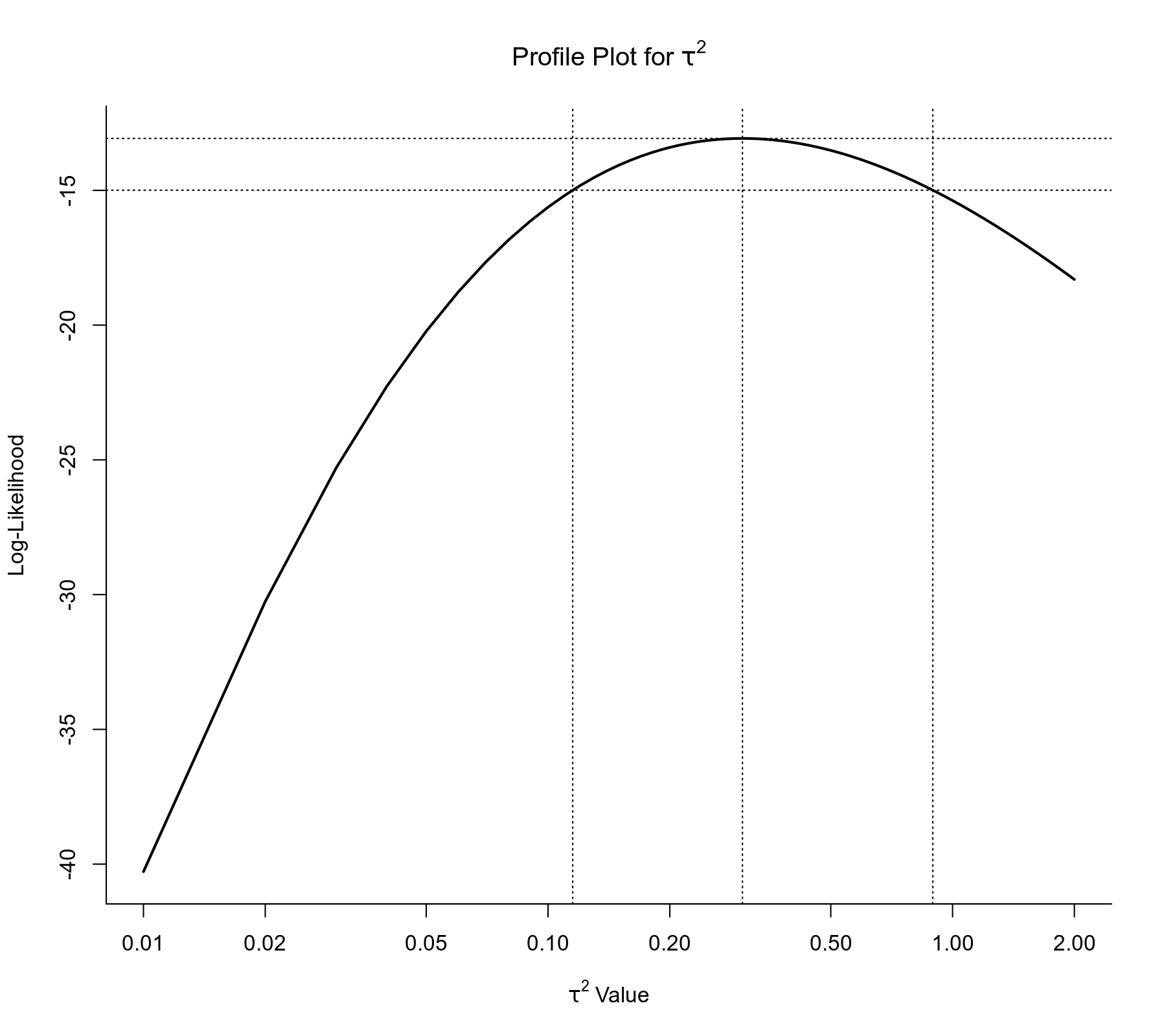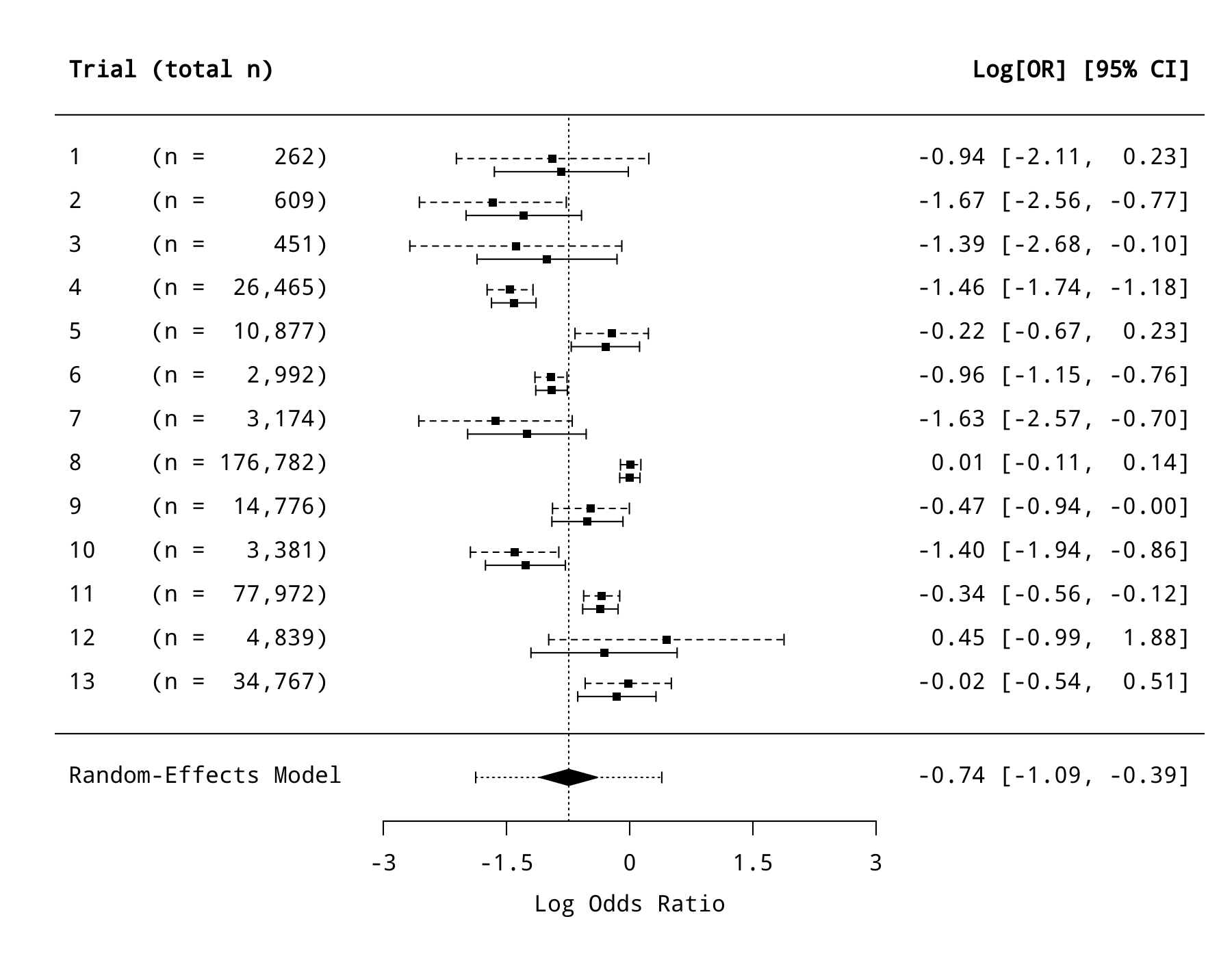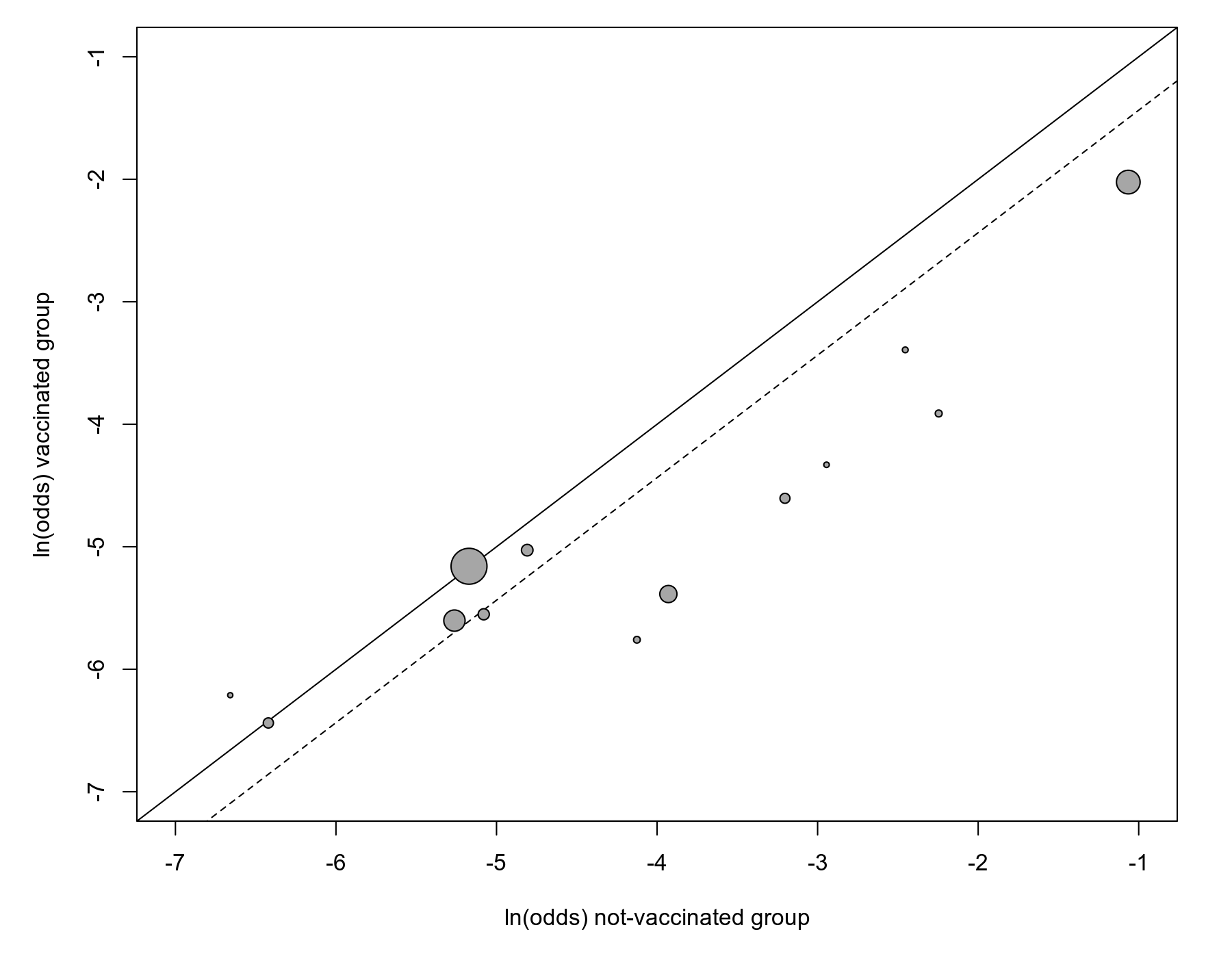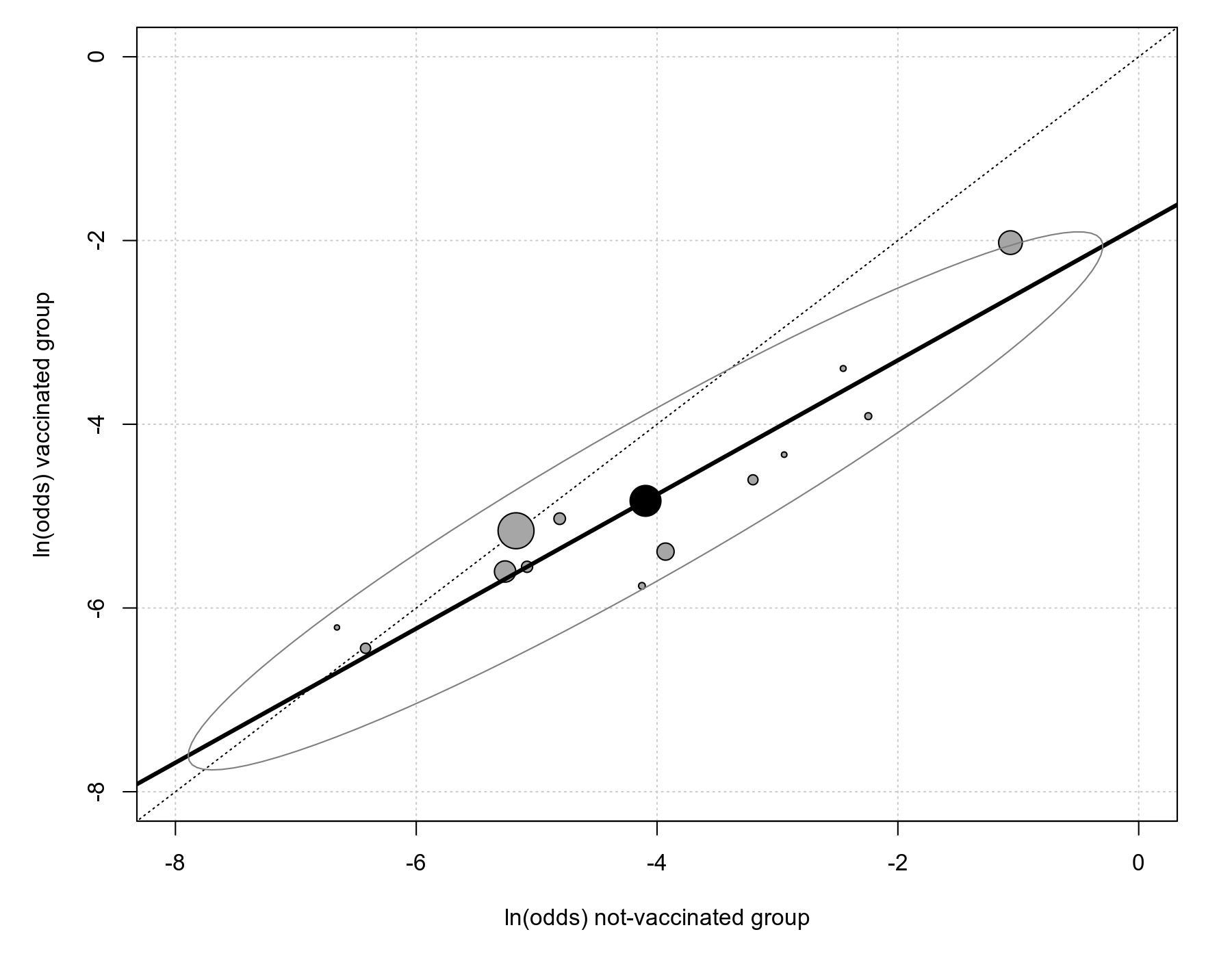#The metafor Package

A Meta-Analysis Package for R

### Site Tools

analyses:vanhouwelingen2002

## van Houwelingen et al. (2002)

### The Methods and Data

The article by van Houwelingen et al. (2002) is a sequel to the introductory article by Normand (1999) on methods for meta-analysis and focuses on more advanced techniques, such as meta-regression and multivariate models. The authors mostly use SAS throughout the article for fitting the various models. The analyses are replicated here using R.

In the first part of the article, the models and methods are illustrated with data from 13 studies examining the effectiveness of the Bacillus Calmette-Guerin (BCG) vaccine for preventing tuberculosis (Colditz et al., 1994). The data are provided in Table I (p. 594) and can be loaded with:

library(metafor)
dat <- dat.colditz1994
dat

(I copy the dataset into dat, which is a bit shorter and therefore easier to type further below). Variables tpos and tneg in this dataset indicate the number of individuals that were TB positive and negative in the vaccinated (treatment) group, while cpos and cneg indicate the number of individuals that were TB positive and negative in the non-vaccinated (control) group.

We can calculate the individual log odds ratios and corresponding sampling variances with:

dat <- escalc(measure="OR", ai=tpos, bi=tneg, ci=cpos, di=cneg, data=dat)

In addition, we can recode the year variable as in the table with:

dat$year <- dat$year - 1900

The contents of the dataset are then:

   trial               author year tpos  tneg cpos  cneg ablat      alloc      yi     vi
1      1              Aronson   48    4   119   11   128    44     random -0.9387 0.3571
2      2     Ferguson & Simes   49    6   300   29   274    55     random -1.6662 0.2081
3      3      Rosenthal et al   60    3   228   11   209    42     random -1.3863 0.4334
4      4    Hart & Sutherland   77   62 13536  248 12619    52     random -1.4564 0.0203
5      5 Frimodt-Moller et al   73   33  5036   47  5761    13  alternate -0.2191 0.0520
6      6      Stein & Aronson   53  180  1361  372  1079    44  alternate -0.9581 0.0099
7      7     Vandiviere et al   73    8  2537   10   619    19     random -1.6338 0.2270
8      8           TPT Madras   80  505 87886  499 87892    13     random  0.0120 0.0040
9      9     Coetzee & Berjak   68   29  7470   45  7232    27     random -0.4717 0.0570
10    10      Rosenthal et al   61   17  1699   65  1600    42 systematic -1.4012 0.0754
11    11       Comstock et al   74  186 50448  141 27197    18 systematic -0.3408 0.0125
12    12   Comstock & Webster   69    5  2493    3  2338    33 systematic  0.4466 0.5342
13    13       Comstock et al   76   27 16886   29 17825    33 systematic -0.0173 0.0716

### Equal-Effects Model

The first model considered is the equal-effects model based on the log odds ratios. The same model can be fitted with:

res <- rma(yi, vi, data=dat, method="EE")
res
Equal-Effects Model (k = 13)

I^2 (total heterogeneity / total variability):   92.65%
H^2 (total variability / sampling variability):  13.60

Test for Heterogeneity:
Q(df = 12) = 163.1649, p-val < .0001

Model Results:

estimate      se      zval    pval    ci.lb    ci.ub
-0.4361  0.0423  -10.3190  <.0001  -0.5190  -0.3533  ***

---
Signif. codes:  0 '***' 0.001 '**' 0.01 '*' 0.05 '.' 0.1 ' ' 1

For easier interpretation, these results can be back-transformed to the odds ratio scale with:

predict(res, transf=exp, digits=3)
  pred ci.lb ci.ub
0.647 0.595 0.702

These results correspond to those reported on pages 595-596.

### Random-Effects Model

A random-effects model can be fitted (using maximum likelihood estimation) to the same data with:

res <- rma(yi, vi, data=dat, method="ML")
res
Random-Effects Model (k = 13; tau^2 estimator: ML)

tau^2 (estimated amount of total heterogeneity): 0.3025 (SE = 0.1549)
tau (square root of estimated tau^2 value):      0.5500
I^2 (total heterogeneity / total variability):   91.23%
H^2 (total variability / sampling variability):  11.40

Test for Heterogeneity:
Q(df = 12) = 163.1649, p-val < .0001

Model Results:

estimate       se     zval     pval    ci.lb    ci.ub
-0.7420   0.1780  -4.1694   <.0001  -1.0907  -0.3932      ***

---
Signif. codes:  0 ‘***’ 0.001 ‘**’ 0.01 ‘*’ 0.05 ‘.’ 0.1 ‘ ’ 1

Again, we can back-transform these results to the odds ratio scale:

predict(res, transf=exp, digits=3)
  pred ci.lb ci.ub pi.lb pi.ub
0.476 0.336 0.675 0.153 1.478

These results correspond to those reported on pages 597-598.

Figure 1 in the article provides a plot of the profile likelihood function of the between-trial variance (i.e., $\tau^2$). The same figure can be obtained with:

profile(res, xlim=c(0.01,2), steps=100, log="x", cex=0, lwd=2, cline=TRUE)
abline(v=c(0.1151, 0.8937), lty="dotted")The bounds of the 95% profile likelihood based confidence interval (CI) for $\tau^2$ are also added to the figure (i.e., 0.1151 to 0.8937). By default, the confint() function in the metafor package computes CIs for $\tau^2$ using the Q-profile method (Viechtbauer, 2007), but we can also obtain the profile likelihood CI with:1)

confint(res, type="PL")
       estimate   ci.lb   ci.ub
tau^2    0.3025  0.1151  0.8937
tau      0.5500  0.3393  0.9454
I^2(%)  91.2283 79.8303 96.8485
H^2     11.4003  4.9579 31.7310

Constructing a profile likelihood plot (Figure 2 in the article) and corresponding CI for the mean treatment effect (i.e., $\mu$) can also be done, but requires a bit more manual work (see p. 598). As an alternative, I would suggest using the Knapp and Hartung method (Hartung & Knapp, 2001a, 2001b) for constructing the CI for $\mu$, as it achieves essentially nominal coverage in most circumstances (e.g., Hartung & Knapp, 2001a, 2001b; Sánchez-Meca & Marín-Martínez, 2008). This CI can be obtained with:

res <- rma(yi, vi, data=dat, method="ML", test="knha")
predict(res, transf=exp, digits=3)
  pred ci.lb ci.ub pi.lb pi.ub
0.476 0.318 0.714 0.134 1.687

This CI (i.e., 0.318 to 0.714) is just a bit wider than the profile likelihood CI reported in the paper (i.e., 0.323 to 0.691).

Figure 3 in the paper shows the observed log odds ratios with 95% CIs and the corresponding empirical Bayes estimates with 95% posterior confidence intervals. With a bit of work, the same figure can be created with:

res <- rma(yi, vi, data=dat, method="ML")
sav <- blup(res)

par(mar=c(5,5,1,2))
forest(res, refline=res$b, addcred=TRUE, xlim=c(-7,7), alim=c(-3,3), slab=1:13, psize=0.8, ilab=paste0("(n = ", formatC(apply(dat[,c(4:7)], 1, sum), width=7, big.mark=","), ")"), ilab.xpos=-3.5, ilab.pos=2, rows=13:1+0.15, header="Trial (total n)", lty="dashed") arrows(sav$pi.lb, 13:1 - 0.15, sav$pi.ub, 13:1 - 0.15, length=0.035, angle=90, code=3) points(sav$pred, 13:1 - 0.15, pch=15, cex=0.8)The prediction interval shown at the bottom (as the dotted line going through the summary polygon) is slightly wider than the one reported in the article, as it also takes the uncertainty in $\hat{\mu}$ into consideration. This interval (i.e., -1.875 to 0.391) can also be obtained with:

predict(res, digits=3)
   pred    se  ci.lb  ci.ub  pi.lb pi.ub
-0.742 0.178 -1.091 -0.393 -1.875 0.391

### Bivariate Approach

In the next part of the article, the authors introduce the bivariate model for meta-analysis. For this purpose, the dataset is treated in a different way, with each study contributing two data points to the analysis, namely the outcome (i.e., log odds) of the vaccinated (treatment) group and the outcome (i.e., log odds) of the non-vaccinated (control) group. The L'Abbé plot makes this idea explicit (Figure 4 in the paper):

res <- rma(measure="OR", ai=tpos, bi=tneg, ci=cpos, di=cneg, data=dat, method="ML")
labbe(res, xlim=c(-7,-1), ylim=c(-7,-1),
xlab="ln(odds) not-vaccinated group", ylab="ln(odds) vaccinated group")

The dashed line indicates the estimated effect based on the model.The dataset in the corresponding long format can be obtained with:

dat.long <- to.long(measure="OR", ai=tpos, bi=tneg, ci=cpos, di=cneg, data=dat.colditz1994)

The arm-specific outcomes (i.e., log odds) with corresponding sampling variances can be added to the dataset with:

dat.long <- escalc(measure="PLO", xi=out1, mi=out2, data=dat.long)
dat.long$tpos <- dat.long$tneg <- dat.long$cpos <- dat.long$cneg <- NULL
levels(dat.long$group) <- c("CON", "EXP") dat.long  trial author year ablat alloc study group out1 out2 yi vi 1 1 Aronson 1948 44 random 1 EXP 4 119 -3.3928 0.2584 2 1 Aronson 1948 44 random 1 CON 11 128 -2.4541 0.0987 3 2 Ferguson & Simes 1949 55 random 2 EXP 6 300 -3.9120 0.1700 4 2 Ferguson & Simes 1949 55 random 2 CON 29 274 -2.2458 0.0381 5 3 Rosenthal et al 1960 42 random 3 EXP 3 228 -4.3307 0.3377 6 3 Rosenthal et al 1960 42 random 3 CON 11 209 -2.9444 0.0957 7 4 Hart & Sutherland 1977 52 random 4 EXP 62 13536 -5.3860 0.0162 8 4 Hart & Sutherland 1977 52 random 4 CON 248 12619 -3.9295 0.0041 9 5 Frimodt-Moller et al 1973 13 alternate 5 EXP 33 5036 -5.0279 0.0305 10 5 Frimodt-Moller et al 1973 13 alternate 5 CON 47 5761 -4.8087 0.0215 11 6 Stein & Aronson 1953 44 alternate 6 EXP 180 1361 -2.0230 0.0063 12 6 Stein & Aronson 1953 44 alternate 6 CON 372 1079 -1.0649 0.0036 13 7 Vandiviere et al 1973 19 random 7 EXP 8 2537 -5.7593 0.1254 14 7 Vandiviere et al 1973 19 random 7 CON 10 619 -4.1255 0.1016 15 8 TPT Madras 1980 13 random 8 EXP 505 87886 -5.1592 0.0020 16 8 TPT Madras 1980 13 random 8 CON 499 87892 -5.1713 0.0020 17 9 Coetzee & Berjak 1968 27 random 9 EXP 29 7470 -5.5514 0.0346 18 9 Coetzee & Berjak 1968 27 random 9 CON 45 7232 -5.0796 0.0224 19 10 Rosenthal et al 1961 42 systematic 10 EXP 17 1699 -4.6046 0.0594 20 10 Rosenthal et al 1961 42 systematic 10 CON 65 1600 -3.2034 0.0160 21 11 Comstock et al 1974 18 systematic 11 EXP 186 50448 -5.6030 0.0054 22 11 Comstock et al 1974 18 systematic 11 CON 141 27197 -5.2621 0.0071 23 12 Comstock & Webster 1969 33 systematic 12 EXP 5 2493 -6.2118 0.2004 24 12 Comstock & Webster 1969 33 systematic 12 CON 3 2338 -6.6584 0.3338 25 13 Comstock et al 1976 33 systematic 13 EXP 27 16886 -6.4384 0.0371 26 13 Comstock et al 1976 33 systematic 13 CON 29 17825 -6.4211 0.0345 Since there is no overlap in the data used to calculate these arm-specific outcomes, the observed outcomes are (conditionally) independent. However, the corresponding true outcomes are likely to be correlated. The bivariate model allows us to estimate the variance and correlation of the true outcomes in the two arms: res <- rma.mv(yi, vi, mods = ~ group - 1, random = ~ group | trial, struct="UN", data=dat.long, method="ML") res Multivariate Meta-Analysis Model (k = 26; method: ML) Variance Components: outer factor: trial (nlvls = 13) inner factor: group (nlvls = 2) estim sqrt k.lvl fixed level tau^2.1 2.4073 1.5516 13 no CON tau^2.2 1.4314 1.1964 13 no EXP rho.CON rho.EXP CON EXP CON 1 0.9467 - no EXP 0.9467 1 13 - Test for Residual Heterogeneity: QE(df = 24) = 5270.3863, p-val < .0001 Test of Moderators (coefficient(s) 1:2): QM(df = 2) = 292.4633, p-val < .0001 Model Results: estimate se zval pval ci.lb ci.ub groupCON -4.0960 0.4347 -9.4226 <.0001 -4.9480 -3.2440 *** groupEXP -4.8337 0.3396 -14.2329 <.0001 -5.4994 -4.1681 *** --- Signif. codes: 0 ‘***’ 0.001 ‘**’ 0.01 ‘*’ 0.05 ‘.’ 0.1 ‘ ’ 1 These are the same results are shown on page 604. We can directly obtain the estimated (mean) log odds ratio from this model by not removing the intercept: res <- rma.mv(yi, vi, mods = ~ group, random = ~ group | trial, struct="UN", data=dat.long, method="ML") The last part of the output is then: Model Results: estimate se zval pval ci.lb ci.ub intrcpt -4.0960 0.4347 -9.4226 <.0001 -4.9480 -3.2440 *** groupEXP -0.7378 0.1797 -4.1047 <.0001 -1.0901 -0.3855 *** The estimate of$\mu$(i.e.,$-0.74$) is essentially the same as the one obtained earlier based on the random-effects model of the (log) odds ratios. Also, based on the bivariate model, we can estimate the amount of heterogeneity in the true log odds ratios with: round(res$tau2 + res$tau2 - 2*res$rho*sqrt(res$tau2*res$tau2), 3)
 0.324

This value is quite close to the estimate of $\tau^2$ obtained earlier.

### Regression of True Log Odds

The results above show that the underlying true log odds in the vaccinated and unvaccinated groups are strongly correlated ($\hat{\rho} \approx 0.947$). As discussed in the article (p. 601), one can also derive the regression line when regressing the true log odds in the vaccinated on the true log odds in the unvaccinated group based on the results from the bivariate model. The intercept and slope of the regression line can be obtained as follows:

res <- rma.mv(yi, vi, mods = ~ group - 1,
random = ~ group | trial, struct="UN",
data=dat.long, method="ML")
reg <- matreg(y=2, x=1, R=res$G, cov=TRUE, means=coef(res), n=res$g.levels.comb.k)
reg
         estimate      se     tval  df    pval    ci.lb    ci.ub
intrcpt   -1.8437  0.3265  -5.6477  11  0.0001  -2.5623  -1.1252  ***
CON        0.7300  0.0749   9.7467  11  <.0001   0.5651   0.8948  ***

---
Signif. codes:  0 '***' 0.001 '**' 0.01 '*' 0.05 '.' 0.1 ' ' 1

Then Figure 5 in the paper (p. 605) can be recreated with:

tmp <- rma(measure="OR", ai=tpos, bi=tneg, ci=cpos, di=cneg, data=dat, method="EE")
labbe(tmp, xlim=c(-8,0), ylim=c(-8,0),
xlab="ln(odds) not-vaccinated group", ylab="ln(odds) vaccinated group",
lty=c("dotted", "blank"), grid=TRUE)
points(coef(res), coef(res), pch=19, cex=3)
abline(a=reg$tab$beta, b=reg$tab$beta, lwd=3)

If the ellipse package is installed (install.packages("ellipse")), we can add the 95% coverage region/ellipse with:

library(ellipse)
xy <- ellipse(res$G, centre=coef(res), level=0.95) lines(xy[,1],xy[,2], col="gray50")Note: The ellipse shown in Figure 5 in the paper appears to be drawn incorrectly. In particular, it appear to be too wide along the y-axis. The following code shows that the ellipse drawn above has the correct width for one of the points. x <- c(coef(res), -3.89047055) c(t(x - coef(res)) %*% solve(res$G) %*% (x - coef(res)))
abline(v=x)
abline(h=x)

While the coefficient (i.e., 0.7300) of the relationship between the underlying true log odds in the vaccinated and unvaccinated groups obtained above is correct, the computation of the corresponding standard error is not quite right, as it assumes that the variance-covariance matrix used as input to matreg() (i.e., res$G) was obtained from 'raw data' for res$g.levels.comb.k (i.e., 13) paired data points, when in fact res$G is the estimated variance-covariance matrix of the random effects from the bivariate model. To obtain a more appropriate standard error, we need to first obtain the variance-covariance matrix of the estimates in res$G, which we can get with:2)

res <- rma.mv(yi, vi, mods = ~ group - 1,
random = ~ group | trial, struct="UN",
data=dat.long, method="ML", cvvc="varcov", control=list(nearpd=TRUE))

Now res$vvc contains the variance-covariance matrix of the estimates in res$G:

res$vvc  tau^2.1 cov tau^2.2 tau^2.1 0.9359073 0.6711028 0.4822374 cov 0.6711028 0.5245460 0.4066553 tau^2.2 0.4822374 0.4066553 0.3399395 We can then use this matrix as part of the input to matreg(), that is, instead of specifying the sample size via argument n as was done above, we specify argument V with this matrix as input: matreg(y=2, x=1, R=res$G, cov=TRUE, means=coef(res), V=res$vvc)  estimate se zval pval ci.lb ci.ub intrcpt -1.8437 0.3548 -5.1967 <.0001 -2.5391 -1.1484 *** CON 0.7300 0.0866 8.4276 <.0001 0.5602 0.8998 *** Now the standard error of the coefficient of interest is computed in such a way that it correctly takes the imprecision of the estimates in res$G into consideration.

### Meta-Regression

It may be possible to account for (at least part of) the heterogeneity in the treatments effects (i.e., log odds ratios) based on one or more moderator variables (i.e., study characteristics that may influence the size of the effect). Such analyses are typically conducted with meta-regression models, as described in the article. For example, a meta-regression model which includes the absolute latitude of the study location as a predictor of the treatment effect can be fitted with:

res <- rma(yi, vi, mods = ~ ablat, data=dat, method="ML")
res
Mixed-Effects Model (k = 13; tau^2 estimator: ML)

tau^2 (estimated amount of residual heterogeneity):     0.0040 (SE = 0.0086)
tau (square root of estimated tau^2 value):             0.0634
I^2 (residual heterogeneity / unaccounted variability): 9.71%
H^2 (unaccounted variability / sampling variability):   1.11
R^2 (amount of heterogeneity accounted for):            98.67%

Test for Residual Heterogeneity:
QE(df = 11) = 25.0954, p-val = 0.0088

Test of Moderators (coefficient(s) 2):
QM(df = 1) = 94.0098, p-val < .0001

Model Results:

estimate      se     zval    pval    ci.lb    ci.ub
intrcpt    0.3710  0.1061   3.4963  0.0005   0.1630   0.5789  ***
ablat     -0.0327  0.0034  -9.6959  <.0001  -0.0393  -0.0261  ***

---
Signif. codes:  0 ‘***’ 0.001 ‘**’ 0.01 ‘*’ 0.05 ‘.’ 0.1 ‘ ’ 1

The same results are given on page 608.

Models with publication year, method of allocation, and the model including absolute latitude and publication year as predictors can be fitted with (output not shown):

rma(yi, vi, mods = ~ year, data=dat, method="ML")
rma(yi, vi, mods = ~ alloc, data=dat, method="ML")
rma(yi, vi, mods = ~ ablat + year, data=dat, method="ML")

The bivariate meta-regression model can be fitted with:

res <- rma.mv(yi, vi, mods = ~ group + group:I(ablat-33) - 1,
random = ~ group | trial, struct="UN",
data=dat.long, method="ML")
res
Multivariate Meta-Analysis Model (k = 26; method: ML)

Variance Components:

outer factor: trial (nlvls = 13)
inner factor: group (nlvls = 2)

estim    sqrt  k.lvl  fixed  level
tau^2.1    1.1819  1.0872     13     no    CON
tau^2.2    1.2262  1.1073     13     no    EXP

rho.CON  rho.EXP    CON  EXP
CON        1   1.0000      -   no
EXP   1.0000        1     13    -

Test for Residual Heterogeneity:
QE(df = 22) = 2862.6396, p-val < .0001

Test of Moderators (coefficient(s) 1:4):
QM(df = 4) = 426.2408, p-val < .0001

Model Results:

estimate      se      zval    pval    ci.lb    ci.ub
groupCON                 -4.1174  0.3061  -13.4529  <.0001  -4.7172  -3.5175  ***
groupEXP                 -4.8257  0.3129  -15.4240  <.0001  -5.4389  -4.2125  ***
groupCON:I(ablat - 33)    0.0725  0.0219    3.3057  0.0009   0.0295   0.1154  ***
groupEXP:I(ablat - 33)    0.0391  0.0224    1.7471  0.0806  -0.0048   0.0830    .

---
Signif. codes:  0 ‘***’ 0.001 ‘**’ 0.01 ‘*’ 0.05 ‘.’ 0.1 ‘ ’ 1

The same results are given on pages 612-613. Note that absolute latitude was centered at 33 degrees, as was done by the authors. The model does converge without any difficulties, despite the very high correlation (which is essentially indistinguishable from 1).

The difference in slopes can be directly obtained with:

res <- rma.mv(yi, vi, mods = ~ group*I(ablat-33),
random = ~ group | trial, struct="UN",
data=dat.long, method="ML")

The results are the same as before, except for the last part, which is now equal to:

Model Results:

estimate      se      zval    pval    ci.lb    ci.ub
intrcpt                  -4.1174  0.3061  -13.4529  <.0001  -4.7172  -3.5175  ***
groupEXP                 -0.7083  0.0481  -14.7301  <.0001  -0.8026  -0.6141  ***
I(ablat - 33)             0.0725  0.0219    3.3057  0.0009   0.0295   0.1154  ***
groupEXP:I(ablat - 33)   -0.0333  0.0028  -11.6988  <.0001  -0.0389  -0.0277  ***

### Random-Effects Conditional Logistic Model

On page 616, the authors describe the possibility of analyzing these data with a (random-effects) conditional logistic model. More details on this approach can be found in Stijnen, Hamza, and Ozdemir (2010) and van Houwelingen, Zwinderman, and Stijnen (1993). The results for this model are not given in the paper, but can be obtained with:

res <- rma.glmm(measure="OR", ai=tpos, bi=tneg, ci=cpos, di=cneg, data=dat, model="CM.EL", method="ML")
res
Random-Effects Model (k = 13; tau^2 estimator: ML)
Model Type: Conditional Model with Exact Likelihood

tau^2 (estimated amount of total heterogeneity): 0.3116 (SE = 0.1612)
tau (square root of estimated tau^2 value):      0.5582
I^2 (total heterogeneity / total variability):   91.46%
H^2 (total variability / sampling variability):  11.72

Tests for Heterogeneity:
Wld(df = 12) = 202.1150, p-val < .0001
LRT(df = 12) = 176.8738, p-val < .0001

Model Results:

estimate      se     zval    pval    ci.lb    ci.ub
-0.7538  0.1801  -4.1862  <.0001  -1.1067  -0.4009  ***

---
Signif. codes:  0 ‘***’ 0.001 ‘**’ 0.01 ‘*’ 0.05 ‘.’ 0.1 ‘ ’ 1

Some patience is required when fitting this model. Since the sample sizes of the individual studies are quite large, the results are very similar to those obtained earlier using the "normal-normal" model.

### Mixture Model

Instead of assuming normally distributed random effects, an alternative analysis approach is to estimate the components of a non-parametric mixture. For more details, see page 617 in the article and the references provided therein. For illustration purposes, the results given in the article can be obtained with the CAMAN package:

library(CAMAN)
res <- mixalg(obs="yi", var="vi", data=dat)
res
Computer Assisted Mixture Analysis:

Data consists of 13 observations (rows).
The Mixture Analysis identified 4 components of a gaussian distribution:

DETAILS:
p         mean
1 0.3552377 -1.457730429
2 0.1505239 -0.967784453
3 0.2979943 -0.329557291
4 0.1962441  0.002256384

Log-Likelihood: -8.324311     BIC: 34.60327

A four-point mixture is obtained. The mean and variance implied by this mixture are:

sum(res@p * res@t)
 -0.7612789

and

sum(res@p * (res@t - sum(res@p*res@t))^2)
 0.348674

respectively. The mean and variance agree quite well with the random-effects model assuming normally distributed true effects.

### Multiple Outcomes

When multiple outcomes are measured based on the same sample of subjects within a study, we can again use a multivariate model for the analysis, but now the correlation among the observed outcomes needs to be accounted for. The authors illustrate such an analysis with the data from Berkey et al. (1998). Please follow the link for more details on analyzing such data (including a reproduction of the results given on pages 617-620).

Berkey, C. S., Hoaglin, D. C., Antczak-Bouckoms, A., Mosteller, F., & Colditz, G. A. (1998). Meta-analysis of multiple outcomes by regression with random effects. Statistics in Medicine, 17(22), 2537–2550.

Colditz, G. A., Brewer, T. F., Berkey, C. S., Wilson, M. E., Burdick, E., Fineberg, H. V., & Mosteller, F. (1994). Efficacy of BCG vaccine in the prevention of tuberculosis: Meta-analysis of the published literature. Journal of the American Medical Association, 271(9), 698–702.

Hartung, J., & Knapp, G. (2001a). A refined method for the meta-analysis of controlled clinical trials with binary outcome. Statistics in Medicine, 20(24), 3875–3889.

Hartung, J., & Knapp, G. (2001b). On tests of the overall treatment effect in meta-analysis with normally distributed responses. Statistics in Medicine, 20(12), 1771–1782.

van Houwelingen, H. C., Arends, L. R., & Stijnen, T. (2002). Advanced methods in meta-analysis: Multivariate approach and meta-regression. Statistics in Medicine, 21(4), 589–624.

van Houwelingen, H. C., Zwinderman, K. H., & Stijnen, T. (1993). A bivariate approach to meta-analysis. Statistics in Medicine, 12(24), 2273–2284.

Sánchez-Meca, J., & Marín-Martínez, F. (2008). Confidence intervals for the overall effect size in random-effects meta-analysis. Psychological Methods, 13(1), 31–48.

Stijnen, T., Hamza, T. H., & Ozdemir, P. (2010). Random effects meta-analysis of event outcome in the framework of the generalized linear mixed model with applications in sparse data. Statistics in Medicine, 29(29), 3046–3067.

Viechtbauer, W. (2007). Confidence intervals for the amount of heterogeneity in meta-analysis. Statistics in Medicine, 26(1), 37–52.

1)
On page 597, the authors show yet another CI for $\tau^2$ that is based on a Satterthwaite approximation (0.1350 to 1.1810). Interestingly, this CI is very close to the one given by confint(res) based on the Q-profile method (0.1302 to 1.1812).
2)
By default, there are numerical problems inverting the Hessian matrix in this example, which we can work around using control=list(nearpd=TRUE).